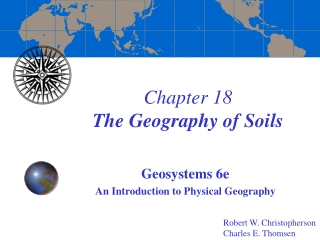DownloadDownload PresentationChapter 18 The Geography of Soils

# Chapter 18 The Geography of Soils

Télécharger la présentation## Chapter 18 The Geography of Soils

- - - - - - - - - - - - - - - - - - - - - - - - - - - E N D - - - - - - - - - - - - - - - - - - - - - - - - - - -
##### Presentation Transcript

1. Chapter 18The Geography of Soils Geosystems 6e An Introduction to Physical Geography Robert W. Christopherson Charles E.Thomsen

2. The Geography of Soils • Soil Characteristics   • Soil Properties   • Soil Formation Factors and Management   • Soil Classification

3. Soil Characteristics • Soil Profiles   • Soil Horizons

4. Soil Sampling and Mapping Units Figure 18.1

5. Mollisol Pedon Profile Figure 18.2

6. Soil Properties • Soil Color   • Soil Texture   • Soil Structure   • Soil Consistence   • Soil Porosity   • Soil Moisture   • Soil Chemistry   • Soil Acidity and Alkalinity

7. Munsell Soil Color Chart Figure 18.3

8. Soil Texture Triangle Figure 18.4

9. Types of Soil Structure Figure 18.5

10. Soil Colloids and CEC Figure 18.6

11. pH Scale Figure 18.7

12. Soil Formation Factors and Management   • Natural Factors   • The Human Factor

14. Soil Classification • Soil Taxonomy   • Diagnostic Soil Horizons   • The 12 Soil Orders of the Soil Taxonomy

15. Soil Taxonomy Figure 18.9

16. Oxisols Figure 18.10

17. Aridisols Figure 18.13

18. Mollisols Figure 18.15

19. Eurasian Soil Fertility Figure 18.16

20. Soils of the Midwest Figure 18.17

21. Alfisols Figure 18.19

22. Ultisols Figure 18.20

23. Spodosols Figure 18.21

24. Entisols Figure 18.22

25. Gelisols Figure 18.23

26. Andisols Figure 18.24

27. Vertisols Figure 18.25

28. Histosols Figure 18.26

29. Soil Contamination in Kesterson Figure FS 18.1.1

30. End of Chapter 18 Geosystems 6e An Introduction to Physical Geography Robert W. Christopherson Charles E.Thomsen